# Lesson Notes By Weeks and Term - Primary 2

Numbers up to 99

TERM: 1st Term

WEEK: 1

CLASS: Primary 2

AGE: 7 years

DURATION: 5 periods of 40 minutes each

DATE:

SUBJECT: Mathematics

TOPIC: Numbers up to 99

SPECIFIC OBJECTIVES: At the end of the lesson, the pupils should be able to

1. Recognise, identify, compare, read and write number symbols and names 0 to 20.
2. Decompose two-digit numbers into multiples of tens and ones and state the value of each digit.
3. Identify the place value of two-digit numbers to 99 and find number patterns.
4. Expanded notation of numbers up to 99; Read and write numbers up to 30.

INSTRUCTIONAL TECHNIQUES: Explanation, question and answer, demonstration, practical

INSTRUCTIONAL MATERIALS: 20 bottle tops per pair of learners, 2 ten frames per learner, number symbol and number name cards, 100 board per learner, 1 hundred and 10 printed tens for the teacher and the learners, 20 bottle tops per learner, place value table per learner, 100 board, ten frames and bottle tops, place value table per learner

PERIOD 1: Numbers up to 20

 PRESENTATION TEACHER’S ACTIVITY PUPIL’S ACTIVITY STEP 1MENTAL MATHS The teacher begins the lesson with some mental calculationsWhat are the next two numbers after1 7, 8, 9...  2 11, 12, 13...  3 20, 19, 18...  4 8, 7, 6...  5 2, 4, 6...  6 0, 5….  7 11, 10….8 10, 12...9 5, 10….10 14, 16… Pupils respond and participate STEP 2CONCEPTDEVELOPMENT The teacher• Asks the learners to count how many bottle tops they each have? (20)• Counts in ones with the learners.Asks the learners questions such as what numbercomes after 2(3) and what number comes before 7 (6)• Revises with the learners that instead of counting in 1s we could also count the bottle tops in 2s.• Asks the learners to put the bottle tops into pairs (2s) and count in 2s forwards from 0 to 20.• Counts backwards in 2s with the learners. (You may wish to revise the terms backwardsand forwards.)• Lastly, asks the learners to put the bottle tops into groups of 5.• Asks them how many groups of 5 they have. (4)• Counts with the learners in 5s, pointing at the groups. Practice counting forwards andbackwards in 5 to 20 with the learners. CLASS ACTIVITYThe teacher• Asks learners to make a group of five bottle tops.• Then asks one learner to make a group of more than five bottle tops• Discusses what learners have done – allow them to speak about it using mathematicallanguage. (I laid out 6 bottle tops. I have one more than 5 bottle tops.)• Asks another learner to make a group of less than five bottle tops.• Discusses what learners have done – allow them to speak about it using mathematicallanguage. (I laid out 3 bottle tops. I have 2 less than 5 bottle tops.)• Writes the number symbols and the number name on the board as learners make the groups and talk about what they have done.• Repeats the activity using numbers between 10 and 20. Lay out 15 bottle tops, then more than and then less than 15.• Discusses what learners have done – allow them to speak about it using mathematicallanguage. (I laid out 18 bottle tops. I have 3 more than 15 bottle tops. I laid out 11 bottle tops. I have 4 less than 15 bottle tops.)• Writes the number symbols on the board as learners make the groups and talk about what they have done. ACTVITY IIThe teacher• Gives each learner 2 ten frames.• Lets them cut out their own ten frames. While they are cutting out the ten frames encourage them to count the blocks on the frame and think about what they could do with a ten frame.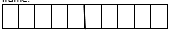• Discusses what you can do with a ten frame with the learners: The ten frame is a concrete aid to use while you learn about the base- ten system. You will use bottle tops when you work with the ten frame. The bottle tops are ones and ten ones make a ten. The thick line shows the middle of the ten frame – it divides the ten frame into 2 halves– there are 5 places on either side of the middle line.• Writes number symbols 0 to 20 and number names one to twenty on the board (or use number symbol and number name cards).• Asks learners to show the number 1 with bottle tops in ten frames. The learners will place one bottle top on the ten frame.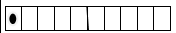• Asks the learners to find the number name for 1 on the board.• Does the same with numbers 2 to 20.You need 2 ten frames to show numbers that are bigger than 10. For example to show 16 you must put 16 bottle tops on the ten frames):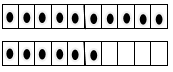• Asks the learners to show any number between 0 and 20 on their ten frames. Asks these numbers randomly.• Asks the learners to identify which number is smaller and which is greater?For example, which of these numbers is smaller than 16 – 6 / 12 / 20 / 1 / 15?• Probes learners’ answers when they make a mistake.• Asks questions such as, why do you think it is smaller than…?Is the number made of tens and ones? How many tens and how many ones?Think again about your answer … which is the smaller number…?Which of these numbers is greater than 16 – 12 / 2 / 19 / 13 / 9?• Probes learners’ answers when they make a mistake.• Asks questions such as, why do you think it is greater than…?Is the number made of tens and ones? How many tens and how many ones?Think again about your answer … which is the bigger number…?• Does this activity using different pairs of numbers. Pupils pay attention and participate STEP 3CLASS-WORK Note: Learners should use their ten frames and bottle tops when they work through this activity.1 Write the following as numbers:a fiveb tenc sixteend twenty 2 Write the number name for 17.3 Which number is smaller? 18 or 13?4 Which number is greater? 11 or 17?5 Write the numbers from smallest to biggest: 15, 12, 14, 11, 13. Pupils attempt their class work STEP 4HOME-WORK 1 Write eighteen as a number symbol.2 Write 14 as a number name.3 Which number is smaller? 20 or 12?4 Write the numbers from biggest to smallest: 15, 12, 14, 11, 13. The pupils writes it in their homework book STEP 5SUMMARY The teacher summarizes by reminding the pupils that we explored numbers up to 20 using our ten frames and bottle tops by comparing the sizes of numbers. She marks their class works, makes corrections where necessary and commends them positively

PERIOD 2: Numbers up to 99

 PRESENTATION TEACHER’S ACTIVITY PUPIL’S ACTIVITY STEP 1MENTAL MATHS The teacher begins the lesson with some mental calculationsFill in the missing ten or one1 15 = 10 + __  2 12 = __ + 2  3 10 = 10 + __  4 14 = __ + 4  5 15 = __ + 5  6 14 = 10 + __7 12 = __ + 28 14 = 10 + __9 18 = 10 + __10 16 = __ + 6 Pupils respond and participate STEP 2CONCEPTDEVELOPMENT The teacher• Reminds the learners that you showed them how to use ten frames in lesson 1. Today you will demonstrate with ten frames because you are going to do other base ten kit activities as well.• Pastes her own large 2 ten frames on the board so that all the learners can see them.• Writes the number 14 on the chalkboard. Asks: How can we make 14 using our bottle tops and ten frames? (Works through setting up this display with the whole class.)• Leads the discussion by saying let’s count out 14 bottle tops.Now that we have 14 bottle tops let’s put them on our ten frames. Let the learners help you to do this.• Asks them: How many groups of ten did we make? (one)Did you have any left over? (Yes 4) We call these four ones.• Asks: How many more do I need to add to the 4 ones to get 10?• Places the bottle tops on the ten frames.• Revises by saying 14 is 1 ten and 4 ones.• Repeats this activity with the following numbers: 17, 19, 13 and 12. Call on different learners to assist in the demonstration so that they participate in the activity. CLASS ACTIVITYThe teacher• Gives each pair a 100 board and 20 bottle tops.• Asks each pair to put a bottle top on 5, 10, 15, 20. Ask the learners what are they counting in?(They are counting in 5s)• Asks each pair to put a bottle top on 10, 20, 30 40, 50. Ask the learners what are they counting in?(They are counting in 10s)• Asks the learners if they notice anything? (10 and 20 are in both the 5s and 10s) ACTIVITY IIThe teacher• Puts 10 ten frames next to the hundred board and alongside each other on the board as shown below. Learners can match their tens onto the columns/rows in the 100 boardyou have given them.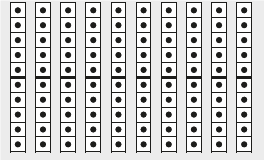As you put (perhaps using prestik) each ten frame on the board, let learners count in 10s.• Shows the learners that 10 tens is the same as 100, by matching the 10 tens onto the printed hundred.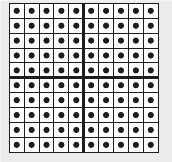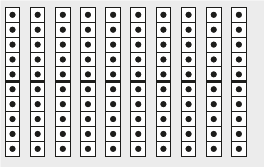Learners must understand the relationship between ones, tens and hundreds. The bottle tops, tens and units in the base ten kit will help them to do this. (Show learners againif necessary that one bottle top is a one, ten bottle tops makes 1 ten, 10 tens makes 1 hundred.)• Writes the numbers 25, 15, 50 and 65 on the board.• Asks a learner to come to the board to show you 25 using the printed 10 frames. Discusses with the learners that 25 is 2 tens and 5 ones. Use the printed tens to count the tens.• Repeats this exercise using 15, 50 and 65. Include different learners each time. Pupils pay attention and participate STEP 3CLASS-WORK Note: Learners should use their base ten kits while they do this classwork.1 Counting on a number board.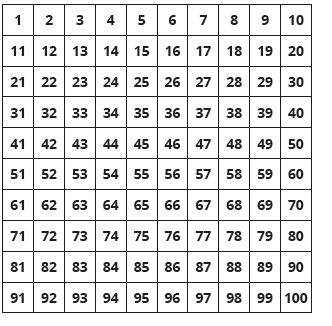2 Write these numbers using tens and ones.a 12b 27c 82d 793 What is 10 more than 11?4 What is 10 less than 17?5 Fill in the missing number: 17 = 10 + _6 Complete the table by writing numbers as tens and ones. The first row is complete.Pupils attempt their class work STEP 4HOME-WORK 1 What is: 5 more than 20?2 What is: 5 less than 30?3 Complete the table by writing numbers as tens and ones. The first row is complete.The pupils writes it in their homework book STEP 5SUMMARY The teacher summarizes by reminding the pupils that found out that in today’s lesson a 100 is equal to 10 tens. I showed this to you on the board when Iput 10 ten frames next to each other to make 100. We also counted backwards and forwards in 5s and 10s to 100. She marks their class works, makes corrections where necessary and commends them positively

PERIOD 3: Numbers up to 99

 PRESENTATION TEACHER’S ACTIVITY PUPIL’S ACTIVITY STEP 1MENTAL MATHS The teacher begins the lesson with some mental calculationsFill in the missing ten or one1 11 = 10 + __2 13 = __ + 3   3 12 = 10 + __    4 18 = __ + 8      5 16 = __ + 6     6 14 = 10 + __7 19 = __ + 98 15 = 10 + __9 17 = 10 + __10 10 = __ + 0 Pupils respond and participate STEP 2CONCEPTDEVELOPMENT The teacher• Gives each learner a 100 board.• Ask the learners to cover the numbers 10, 20, 30, 40, 50, 60, 70, 80, 90 and 100 with bottle tops.• Counts from 0 to 100 in 10s forwards and backwards with the learners. The learners should point at the numbers as they count.• Asks them what pattern do you see? (They are all under each other on thenumber board)• Write 10 and 50 on the board.• Ask the learners which number is bigger (50) and why. (10 is one group of 10 and 50is 5 groups of 10)• Ask the learners to put bottle tops on 5, 10, 15, 20, 25, 30, 35, 40, 45 and 50.• Counts from 0 to 50 in 5s forwards and backwards with the learners. The learners should point at the numbers as they count.• Asks them what pattern do you see? (All the numbers are 5 spaces apart or they end in 5 or 0)• Writes 15 and 35 on the board.• Asks the learners which number is bigger (35) and why? (35 is bigger because it has 3 groups of 10 and 5 ones but 15 has one ten and 5 ones) CLASS ACTIVITYIn this activity we will introduce the learners to the place value table (shown below)• Draws the place value table on the board.You will need 5 ten frames and 7 ones/bottle tops.• Writes the number 17 on the board. Asks: How can I show 17 using the base ten kit?The learners should answer 1 ten and 7 ones.• Using the ten frames lets a learner show the class how this is done. They can paste/stick the correct cuts outs from base ten kit onto the place value table. (One printed ten and 7 bottle tops on an empty ten frame can be used to show the number 17 as shown below.)• Discusses with the learners that 17 is one ten and 7 ones.• Repeats these steps with the numbers 31, 25 and 42 and other 2-digit numbers (up to 99) if there is time. Pupils pay attention and participate STEP 3CLASS-WORK Note: Learners should use their base ten kits while they do this classwork.1 Fill in the missing number:a 13 = 10 + __b 21 = 20 + _c 34 = 30 + __d 26 = 20 + __ 2 Circle the bigger number:a 16 or 22b 20 or 32c 42 or 24d 50 or 35e 18 or 38 3 Circle the smallest number:a 6 or 9b 24 or 25c 32 or 22d 18 or 27e 55 or 35 Pupils attempt their class work STEP 4HOME-WORK 1 What is 10 more than 3? 2 What is: 10 more than 20? 3 Complete the following:a 14 = 10 +b 32 = 30 +c 24 = 20 +d 16 = 10 + The pupils writes it in their homework book STEP 5SUMMARY The teacher summarizes by reminding the pupils that in today’s lesson we have counted in 5 and 10s to 100 and looked for patterns. We started to use theplace value table to show tens and ones. For example, 17 is 10 and 7. She marks their class works, makes corrections where necessary and commends them positively

PERIOD 4: Expanded notation

 PRESENTATION TEACHER’S ACTIVITY PUPIL’S ACTIVITY STEP 1MENTAL MATHS The teacher begins the lesson with some mental calculationsWhat is 10 more than the following1 2        2 40      3 30      4 50      5 20    What is 10 less than the following6 107 208 309 4010 17 Pupils respond and participate STEP 2CONCEPTDEVELOPMENT The teacher• Gives each learner a 100 board.• Writes the number symbols and number names 11 to 30 on the board (or use thenumber symbol and number name cards).• Writes the numbers 11 to 30 in the air.• Asks: Point to the number 11 on the 100 board. 11 is 1 ten and 1 one.• Asks: Point to the number 26 on the 100 board. Explain that the number 26 is madeof 20 and 6.• Asks the learners to find the number name for 26 on the board.• Does the same with numbers 12, 18, 23 and 29.Each time explain the importance of knowing the place value breakdown of the number into tens and ones.For example – 23 is made of 2 ten and 3 ones. Ask learners to tell you how the numbers are made up. CLASS ACTIVITYThe teacher• Draws a place value table on the board and show 26 on it using the base ten kit. (You willneed 2 printed full ten frames and 6 bottle tops.)• Writes the number 26 on the board. Asks: How can I show 26 by tens and ones?The learners should answer 2 tens and 6 ones.• Using the tens lets a learner show the class how this is done. They can paste/stick tens onto the place value table.• Discusses the following questions with the learners: Looking at the number 26a Why are there 2 tens shown? (There are 2 tens in 26.)b Does the 6 mean 6 or 60? (It means 6, or 6 ones)c Does the 2 mean 2 or 20? (It means 2 tens, or 20)• Writes the following numbers on the board: 16, 28 and 41 on the board and repeats the activity questions as you did for the number 26 above.• Selects 3 different learners to let them present these numbers using the base ten kits as shown above.• Discusses the value of each digit with the learners.• Compares the 3 given numbers in terms of which is the biggest, smallest and the reasons for this. (41 is the biggest, then 28, 26, 14. 14 is the smallest. 41 has 4 tens, 14 has 1 ten. Etc.) CLASS ACTIVITYThe teacher• Draws a place value table on the board. Use the base ten kit to show 14. Writes a number sentence to express how to break down 14 into tens and units.(14 = 10 + 4)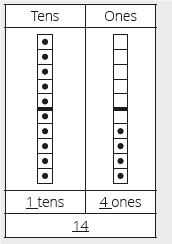• Explains to the learners the relationship between the place value table and the numbersentence. The place value table is a pictorial representation. The number sentence is abstract. It is important that learners recognise the relationship between these.• Repeats this activity using the numbers 18 (10 + 8 = 18) and 16 (10 + 6 = 16) Pupils pay attention and participate STEP 3CLASS-WORK Note: Learners should use their base ten kits while they do this classwork.1 Show the number 15 on the place value table below: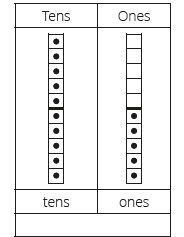2 7 ten + 3 ones = _____3 6 ten + 8 ones = _____4 82 = 80 + _____5 55 = _____ + _____6 90 + _____ = 937 _____ + 6 = 668 _____ + 9 = 59 Pupils attempt their class work STEP 4HOME-WORK 1 7 tens + 5 ones = _____2 5 tens + 0 ones = _____3 67 = _____  tens + _____  ones4 89 = _____  tens + _____  ones The pupils writes it in their homework book STEP 5SUMMARY The teacher summarizes by reminding the pupils how to break numbers down into tens and ones. We also started to add numbers using tens and ones. She marks their class works, makes corrections where necessary and commends them positively

PERIOD 5: Weekly Test/consolidations

TEACHER’S ACTIVITY: The teacher revises all the concepts treated from period 1-4 and gives the pupils follow through exercises, quiz and tests. She marks the exercises, makes corrections and commends the pupils positively.

PUPIL’S ACTVIVITY: The pupils work on the worksheets and exercises give by the teacher individually

CONSOLIDATION

1 Colour 58 circles.

How many tens? ________

How many ones? ________1. Write the answer. The first one has been done for you.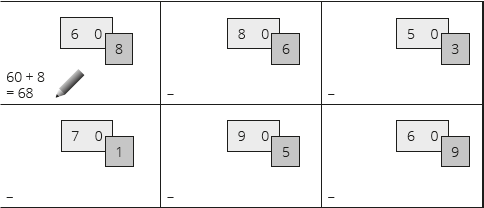1. Complete the following:

a 14 = ____ + ____

b 23 = ____ + ____

c 32 = ____ + ____

d 50 = _____ + ____

e 99 = _____ + ____